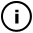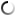# Saturday, December 07, 2013

void setup(){
smooth();
//Makes the umberellas smooth
background(0,0,0);
size(800,800);
frameRate(20);
//slows the frame rate for more inpact.
}
//All this next bit is  about the actual umberellas.
void draw() {
noStroke();
int posx = int(random(0,width));
int posy = int(random(0,height));
//these are the positions which are used
//later to draw the umberellas
fill(random(1,500),random(1,500),random(1,500),30);
strokeWeight(random(0,2));
//here is the code for the circles
//around the star shapes.
pushMatrix();
translate(width/4,height/4);
rotate(PI*count);

//this is the code for the the stars
//in the center of the circles.
for(float i = 0.0; i < 2.0*PI; i+=0.5-radius/200){

}
popMatrix();
if(count<2.1) {
count=count+0.005;
//this code is all about the rotation
}
else{ count=0;}
}
//the end of the code

## infosubmitted by: KeelingAlexandra
views: 42

Tags:

## commentsloading...